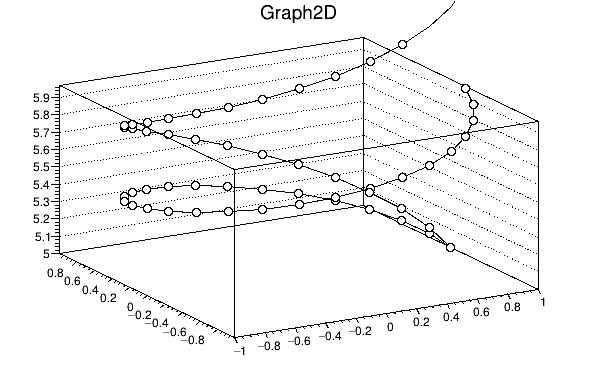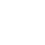# How can I change the z-axis range of a 2d graph

I want to plot a number of 3d curves using TGraph2D since based on searching the forum I found that MultiGraph doesn’t work for 3d curves. I have been able to plot each curve in the same canvas, but the x/y/z plot range is fixed at the values required for the first plot and subsequent plots extend beyond those limits. I tried to change the axis range to accommodate additional plots but that didn’t change the plot. Attached is a test script that I have been using to figure out how to generate the plot. The actual data to be plotted is generated in a Monte Carlo simulation. Does anyone have any thoughts on either a way to change the axis limits or an alternative way to generate this plot?``````#include "TGraph.h"
#include "TAxis.h"
{
double pi=4.*atan(1.);
auto c1 =new TCanvas("c1","Test1",0,0,600,400);
auto g = new TGraph2D();
double x, y, z;
for(int n=0;n<2;n++){
for(int k=0;k<30;k++){
x=cos(2*pi*k/30.);
y=sin(2*pi*k/30.);
z = (n+1)*k/30.;
g->SetPoint(k,x,y,z);
}
if(n==0){
c1->cd();
g->DrawClone("line""p0");
g->GetZaxis()->SetRangeUser(0.,3.);
}
else
{
c1->cd(1);
g->DrawClone("LINE""P0""SAME");
g->GetZaxis()->SetRangeUser(0.,3.);
}
}
}

``````

ROOT Version: 6-28-00
Platform: Ubuntu 22
Compiler: Not Provided

``````      g->SetMinimum(0.); g->SetMaximum(3.); // before drawing
g->DrawClone("line p0"); // the first graph (defines axes)
``````

Many thanks Wile.